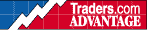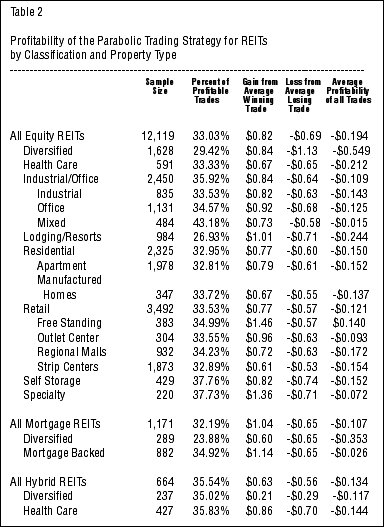HOT TOPICS LIST

INDICATORS LIST

LIST OF TOPICS

# Using Technical Analysis to Manage a REIT Portfolio Part II -- Parabolic

02/14/01 10:27:49 AM
by Dr. Michael J. Seiler

Does the Parabolic technical trading system work in a trending market?

Security:   N/A
Position:   N/A

 The Parabolic trading system, so named because of the shape that results when the system is applied to a chart, is designed for use in trending markets. Once a trend has begun, the system allows for moderate anti-trend movements, but as the trend matures, the system's protective trailing stop (referred to as "stop-and-reverse (SAR)") follows the price movements at a progressively closer rate. Once the SAR is reached, an equal and opposite position is taken in the market. In fact, the Parabolic trading system always has you in the market with either a long or short position. The calculations for the Parabolic trading system are as follows:SARbuy = SAR-1 + AF(Low - SAR-1)SARsell = SAR-1 + AF(High - SAR-1)where,SAR-1 = Stop-and-reverse value from the previous price,SARbuy = Stop-and-reverse price on the buy side,SARsell = Stop-and-reverse price on the sell side,High = the highest price since the initial position has been taken,Low = the lowest price since the initial position has been taken,AF = an acceleration factor.The EasyLanguage code for the Parabolic trading system is posted at the end of Part II. DATA AND RESULTSAs in Part I, I've used all 210 REITs in existence, dividing them up into classifications. The results are as follows:Table 2 shows the results from applying the Parabolic trading system. The failure of this trading system is glaring as only one category of REITs is profitable. A second drawback to using this system to trade REITs is that over twice as many trades are involved as the RSI Oscillator system. This means that not only is the Parabolic system unsuccessful in terms of gross profitability, but you will also be charged more in transaction costs which will further reduce the net profitability. In short, the Parabolic system was a failure across all REITs types, all property types, and all but one sub-property type. Parabolic Code Construction with EasyLanguageI. FunctionInputs: AcclFact(NumericSimple);Variables: Pos(-1), SAR(Close), AF(.02), HighValue(High), LowValue(Low);If CurrentBar = 1 ThenPos = 1Else If CurrentBar > 1 Then BeginIf High > HighValue Then HighValue = High; If Low < LowValue ThenLowValue = Low; If Pos = 1 Then BeginIf Low <= Parabolic ThenPos = -1; {Reverse Pos}EndElse BeginIf High >= Parabolic ThenPos = 1; {Reverse Pos}End; End; If Pos = 1 Then BeginIf Pos <> 1 Then BeginSAR = LowValue;AF = AcclFact;LowValue = Low;HighValue = High; EndElse BeginSAR = SAR + AF * (HighValue - SAR);If HighValue > HighValue AND AF < 0.2 Then AF = AF + MinList(AcclFact, (0.2 - AF)); End;If SAR > Low ThenSAR = Low; If SAR > Low Then SAR = Low; EndElse BeginIf Pos <> -1 Then BeginSAR = HighValue;AF = AcclFact;LowValue = Low;HighValue = High; EndElse BeginSAR = SAR + AF * (LowValue - SAR);If LowValue < LowValue AND AF < 0.2 Then AF = AF + MinList(AcclFact, (0.2 - AF)); End;If SAR < High ThenSAR = High; If SAR < High Then SAR = High; End;Parabolic = SAR; II. IndicatorInputs: AF(.02); value1 = Parabolic(AF);If CurrentBar > 1 ThenPlot1(value1, "Parabolic" ) ElsePlot1(Close, "Parabolic");{Parabolic Alert Criteria}If Close Crosses Over Plot1 Then Alert("Parabolic has issued a bullish alert")ElseIf Close Crosses Under Plot1 ThenAlert("Parabolic has issued a bearish alert");III. Long Exit SignalInputs: Acceleration(.02), FirstBarMultp(1.5);Variables: AF(0), StopPrice(0), MP(0), HighValue(0);MP = MarketPosition;If High > HighValue ThenHighValue = High; If MP = 1 Then BeginIf MP <> 1 Then BeginStopPrice = Low - Average(TrueRange, 3) * FirstBarMultp;AF = Acceleration;HighValue = High; EndElse BeginStopPrice = StopPrice + AF * (HighValue - StopPrice);If HighValue > HighValue AND AF < 0.2 Then AF = AF + MinList(Acceleration, 0.2 - AF); End;If StopPrice > Low ThenStopPrice = Low; End;If MP = 1 ThenExitLong ("PT") Next Bar at StopPrice Stop; IV. Short Exit SignalInputs: Acceleration(.02), FirstBarMult(3);Variables: SAR(0), AF(0), StopPrice(0), MP(0), LowValue(0);MP = MarketPosition;If Low < LowValue ThenLowValue = Low; If MP = -1 Then BeginIf MP <> -1 Then BeginStopPrice = High + Average(TrueRange, 3) * FirstBarMult;AF = Acceleration;LowValue = Low; EndElse BeginStopPrice = StopPrice - AF * (StopPrice - LowValue);If LowValue < LowValue AND AF < 0.2 Then AF = AF + MinList(Acceleration, 0.2 - AF); End;If StopPrice < High ThenStopPrice = High; End;IF MP = -1 ThenExitShort ("PT") Next Bar at StopPrice Stop Part I - The RSI OscillatorPart III - MACD

Dr. Michael J. Seiler

 Title: Professor of Finance Company: Hawaii Pacific University Address: 1132 Bishop Street; Suite 504 Honolulu, HI 96813 Phone # for sales: 808-544-0827 Fax: 808-544-0835 E-mail address: mseiler@hpu.edu Traders' Resource Links Hawaii Pacific University has not added any product or service information to TRADERS' RESOURCE.

 Comments or Questions? Article Usefulness 5 (most useful) 4 3 2 1 (least useful)